﻿ 西平王氏家谱【主编王振江，2017年5月出版】_家谱网

# 西平王氏家谱【主编王振江，2017年5月出版】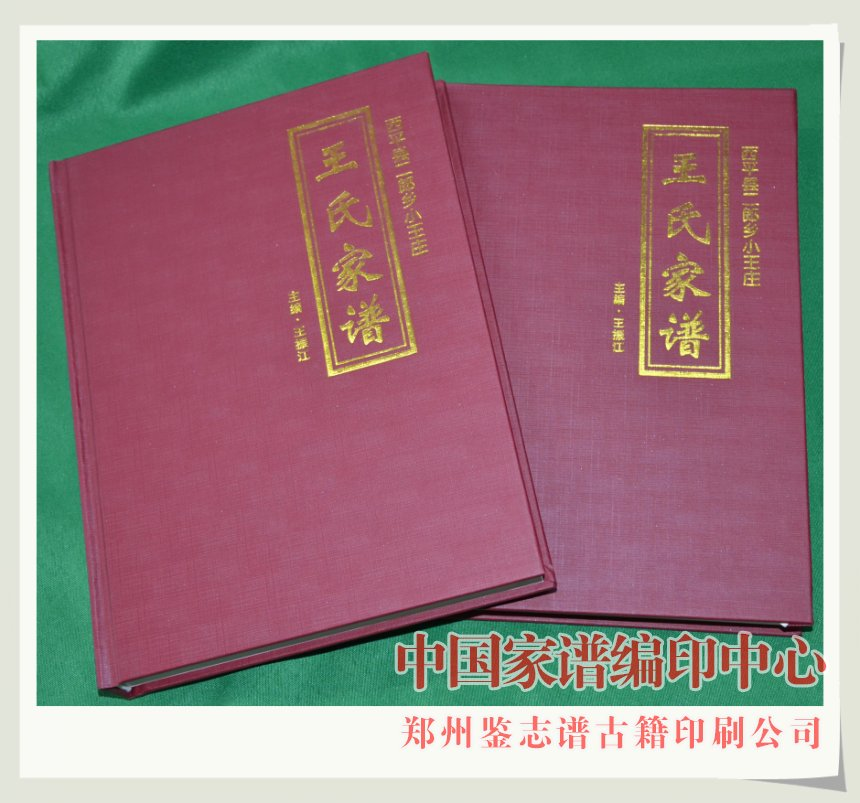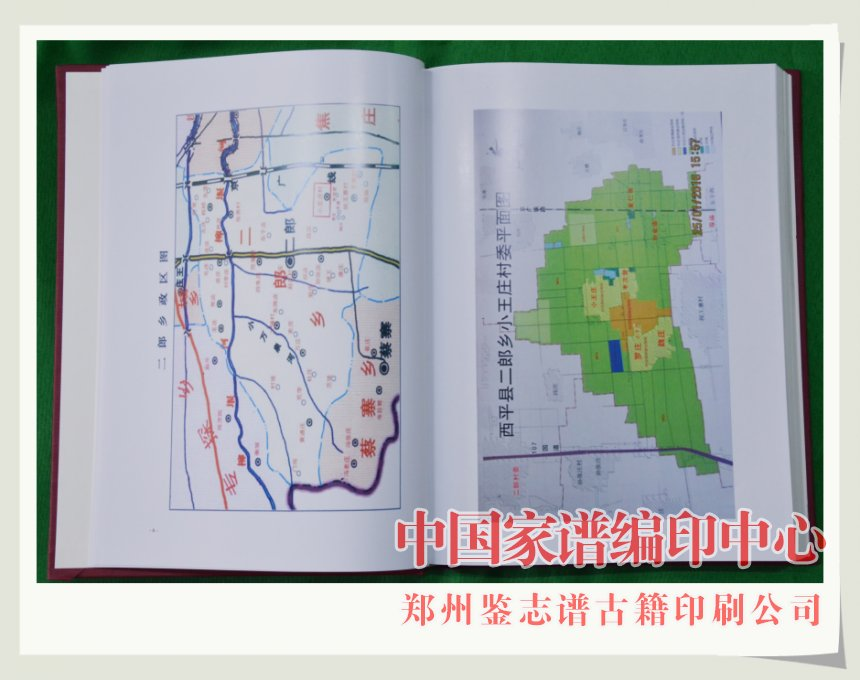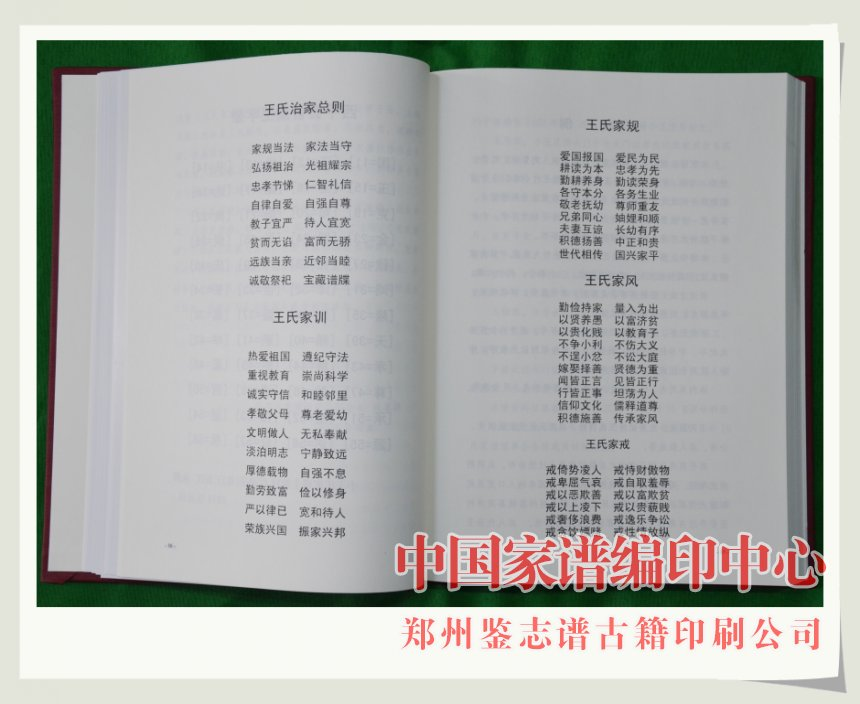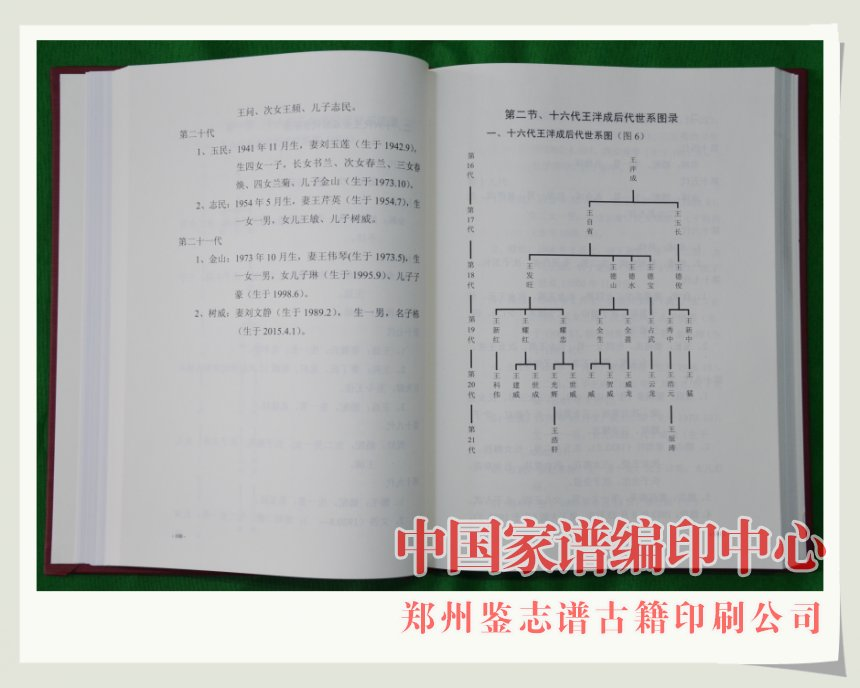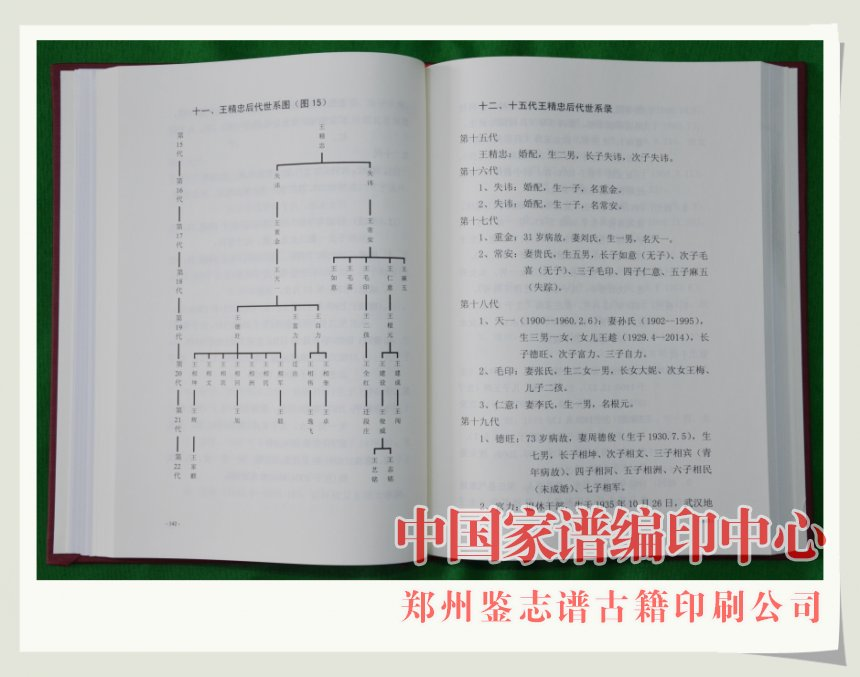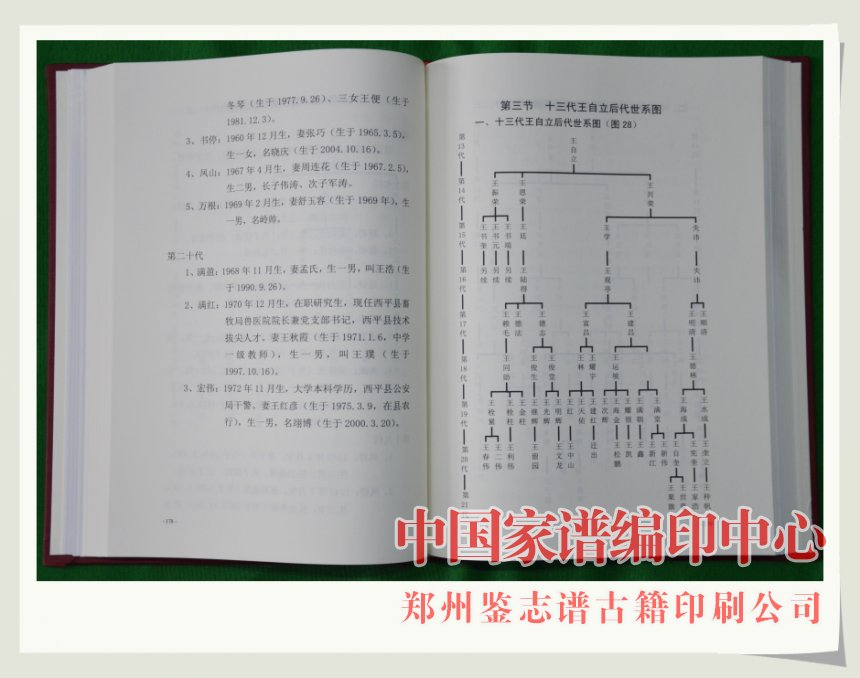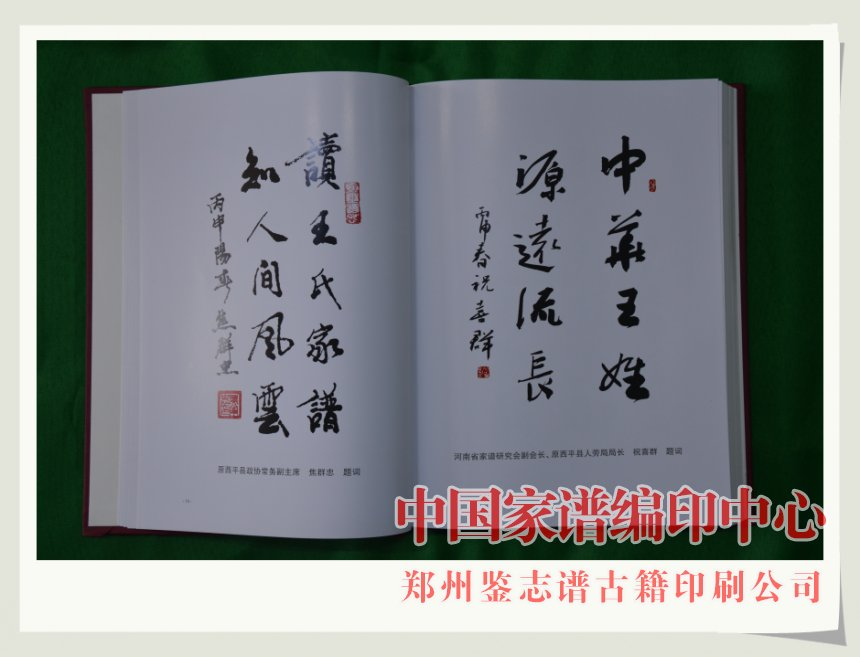[国=11] [正=12] [学=13] [书=14]

[玉=15] [全=16] [逢=17] [选=18]

[克=19] [修=20] [振=21] [良=22]

[金=23] [殿=24] [恩=25] [崇=26]

[钦=27] [化=28] [广=29] [庆=30]

[鸿=31] [泽=32] [世=33] [长=34]

[隆=35] [烈=36] [盛=37] [昌=38]

[天=39] [赐=40] [鹏=41] [举=42]

[孝=43] [廉=44] [略=45] [嘉=46]

[尊=47] [教=48] [博=49] [哲=50]

[承=51] [继=52] [伟=53] [厦=54]

[源=55] [达=56] [获=57] [果=58]

2016年12月16日